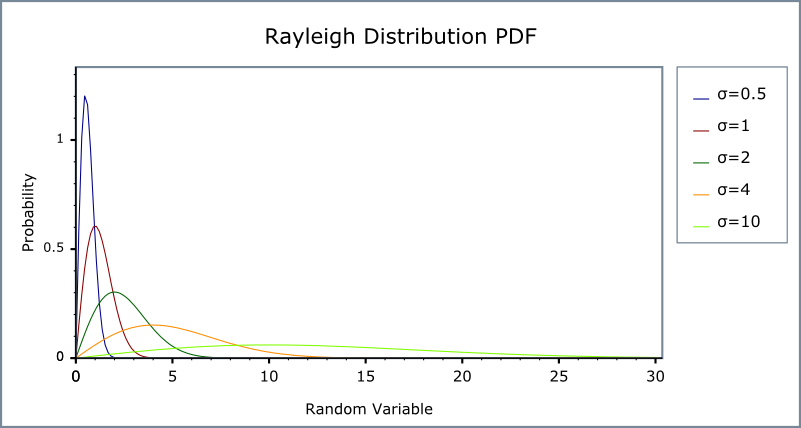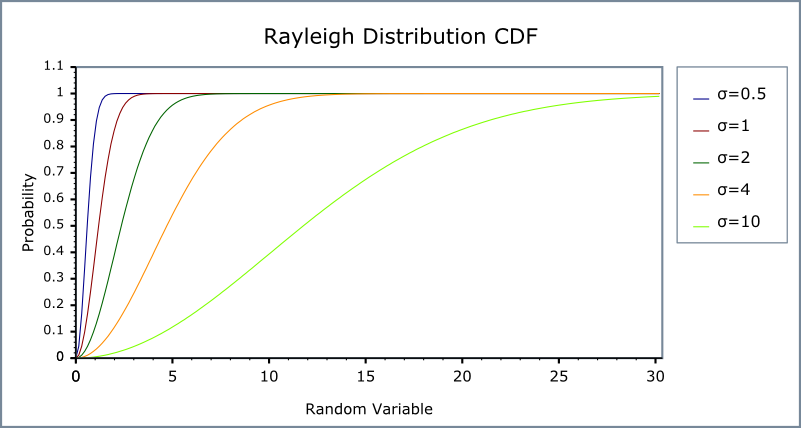#Boost C++ Libraries

...one of the most highly regarded and expertly designed C++ library projects in the world.

#### Rayleigh Distribution

`#include <boost/math/distributions/rayleigh.hpp>`
```namespace boost{ namespace math{

template <class RealType = double,
class Policy   = policies::policy<> >
class rayleigh_distribution;

typedef rayleigh_distribution<> rayleigh;

template <class RealType, class Policy>
class rayleigh_distribution
{
public:
typedef RealType value_type;
typedef Policy   policy_type;
// Construct:
rayleigh_distribution(RealType sigma = 1)
// Accessors:
RealType sigma()const;
};

}} // namespaces
```

The Rayleigh distribution is a continuous distribution with the probability density function:

f(x; sigma) = x * exp(-x2/2 σ2) / σ2

For sigma parameter σ   > 0, and x > 0.

The Rayleigh distribution is often used where two orthogonal components have an absolute value, for example, wind velocity and direction may be combined to yield a wind speed, or real and imaginary components may have absolute values that are Rayleigh distributed.

The following graph illustrates how the Probability density Function(pdf) varies with the shape parameter σ:and the Cumulative Distribution Function (cdf)##### Related distributions

The absolute value of two independent normal distributions X and Y, √ (X2 + Y2) is a Rayleigh distribution.

The Chi, Rice and Weibull distributions are generalizations of the Rayleigh distribution.

##### Member Functions
```rayleigh_distribution(RealType sigma = 1);
```

Constructs a Rayleigh distribution with σ sigma.

Requires that the σ parameter is greater than zero, otherwise calls domain_error.

```RealType sigma()const;
```

Returns the sigma parameter of this distribution.

##### Non-member Accessors

All the usual non-member accessor functions that are generic to all distributions are supported: Cumulative Distribution Function, Probability Density Function, Quantile, Hazard Function, Cumulative Hazard Function, mean, median, mode, variance, standard deviation, skewness, kurtosis, kurtosis_excess, range and support.

The domain of the random variable is [0, max_value].

##### Accuracy

The Rayleigh distribution is implemented in terms of the standard library `sqrt` and `exp` and as such should have very low error rates. Some constants such as skewness and kurtosis were calculated using NTL RR type with 150-bit accuracy, about 50 decimal digits.

##### Implementation

In the following table σ   is the sigma parameter of the distribution, x is the random variate, p is the probability and q = 1-p.

Function

Implementation Notes

pdf

Using the relation: pdf = x * exp(-x2)/2 σ2

cdf

Using the relation: p = 1 - exp(-x2/2) σ2   = -expm1(-x2/2) σ2

cdf complement

Using the relation: q = exp(-x2/ 2) * σ2

quantile

Using the relation: x = sqrt(-2 * σ 2) * log(1 - p)) = sqrt(-2 * σ 2) * log1p(-p))

quantile from the complement

Using the relation: x = sqrt(-2 * σ 2) * log(q))

mean

σ * sqrt(π/2)

variance

σ2 * (4 - π/2)

mode

σ

skewness

kurtosis

kurtosis excess

##### References
 Copyright © 2006-2010, 2012, 2013 Paul A. Bristow, Christopher Kormanyos, Hubert Holin, Bruno Lalande, John Maddock, Johan Råde, Gautam Sewani, Benjamin Sobotta, Thijs van den Berg, Daryle Walker and Xiaogang Zhang Distributed under the Boost Software License, Version 1.0. (See accompanying file LICENSE_1_0.txt or copy at http://www.boost.org/LICENSE_1_0.txt)